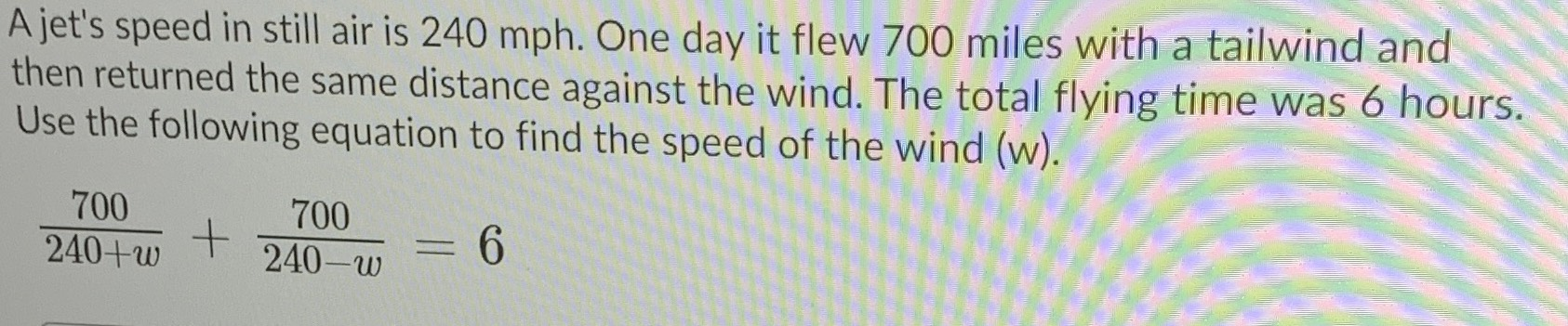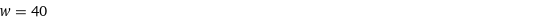### ¿Todavía tienes preguntas de matemáticas?

Pregunte a nuestros tutores expertos
Algebra
PreguntaA jet's speed in still air is $$240 mph$$ . One day it flew $$700$$ miles with a tailwind and then returned the same distance against the wind. The total flying time was $$6$$ hours. Use the following equation to find the speed of the wind (w).

$$\frac { 700 } { 240 + w } + \frac { 700 } { 240 - w } = 6$$